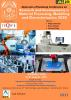# FIRST PLY FAILURE ANALYSIS IN HYBRID COMPOSITE MATERIAL SISAL/E-GLASS FIBER REINFORCED EPOXY RESIN FOR SYMMETRIC LAMINATE

## Authors

S. Sowmya
Department of Mechatronics Engineering, Hindusthan College of Engineering and Technology, Coimbatore, Tamilnadu, India
M. Sindhu Kavi
Department of Mechanical Engineering, SNS College of Technology, Coimbatore, Tamilnadu, India

### Synopsis

The objective of the paper is to analyse the strength of each layer of hybrid composite material sisal/E-glass fiber reinforced epoxy for symmetric laminate which consists of 8 layers. If there is low strength ratio in one layer, maximum load is calculated to that layer. The strength ratio for all the plies/layers in the laminate are done using the maximum strain and Tsai –Wu failure theories. Considering the strength ratio, maximum load applied is calculated to determine the laminate safe using First Ply Failure (FPF) analysis method. First Ply Failure analysis (FPF) is a method to predict the strength of laminate. Conclusively, the results for on axis stress and strain are calculated, then strength ratio of each layer is greater than 1 which means all 8 layers are safe. Minimal value of strength ratio lets say SR2 = 1.81, the maximum load calculated are σxmax= 27.15 MPa, σymax = 54.3 MPa and τxymax = 18.1 MPa are obtained.Published
February 6, 2021